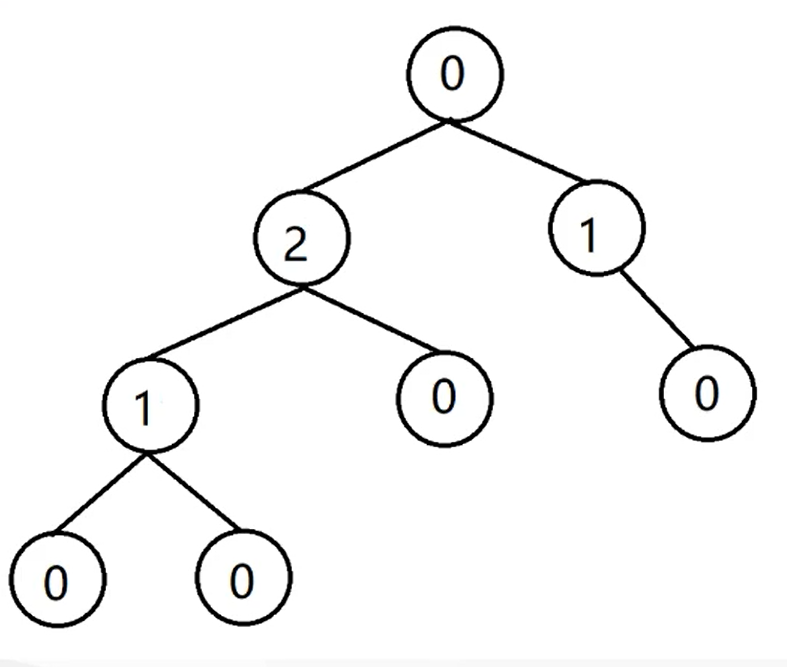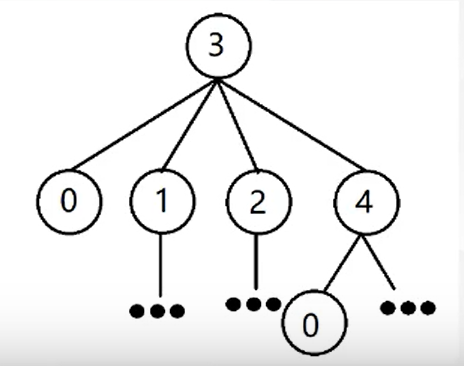# ACM-博弈论基础

## 博弈论基础

#### 博弈论题目特点

• 两名选手，交替进行预先规定好的操作
• 在任何情况下，合法操作只取决于情况本身，与选手无关
• 游戏失败的最终判定往往是选手无法进行合法操作了

## 经典博弈

### 巴什博弈（Bash Game）

``````if (n % (m + 1))  return false;
else  return true;
``````

### 斐波那契博弈（Fibonacci Nim Game）

``````f = f = 1;
for (int i = 0; f[i - 1] < n; i++)
{
f[i] = f[i - 1] + f[i - 2];
if (f[i] == n)  return true;
}
return false;
``````

### 威佐夫博弈（Wythoff Game）

``````double r = (sqrt(5) + 1) / 2;
int d = abs(a - b) * r;
if (d != min(a, b))  return true;
else  false;
``````

### 尼姆博弈（Nimm Game）

``````0001^0010^0011=0000
``````

``````int res = 0;
for (int i = 1; i <= n; i++)
res ^= arr[i];
if (res)  return true;
else  return false;
``````

### SG函数

SG函数（Sprague-Grundy）：对于一个给定的有向无环图，定义关于这个图的每个顶点的SG函数如下：

\[sg(x)=mex{sg(y) | y是x的后继}\]

SG函数的求法：

1. 找出必败态
2. 找出当前所有状态的前驱结点
3. 根据定义计算结点SG值
4. 重复上述步骤，直到整棵树建立完成SG定理：所以我们可以定义有向图游戏的和。设G1,G2,…Gn为n个“有向图”游戏的和(Sum)，游戏G的移动规则是：任选一个子游戏Gi并移动上面的棋子。SG定理就是：sg(G)=sg(G1)∧sg(G2)∧….∧sg(Gn)。也就是说，游戏的SG函数值就是它的所有子游戏的SG函数值的异或。